• 奋战在一线的医护人员，谢谢你们的付出，你们辛苦了！

• 武汉加油！中国加油！# 并非英雄级 永久武器大盘点 下篇

5个月前 (02-21) 135次浏览

\r

\r

\r

\r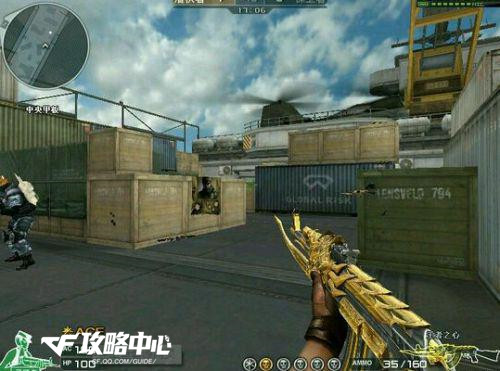\r

\r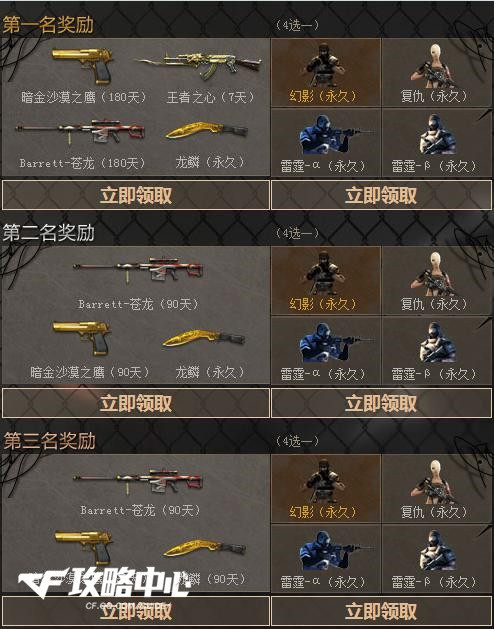\r

\r

\r

\r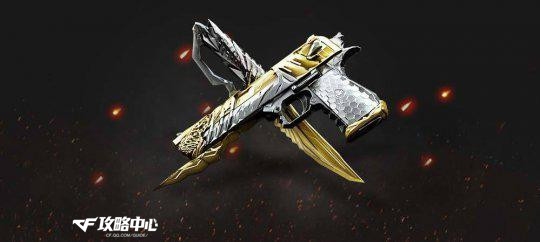\r

\r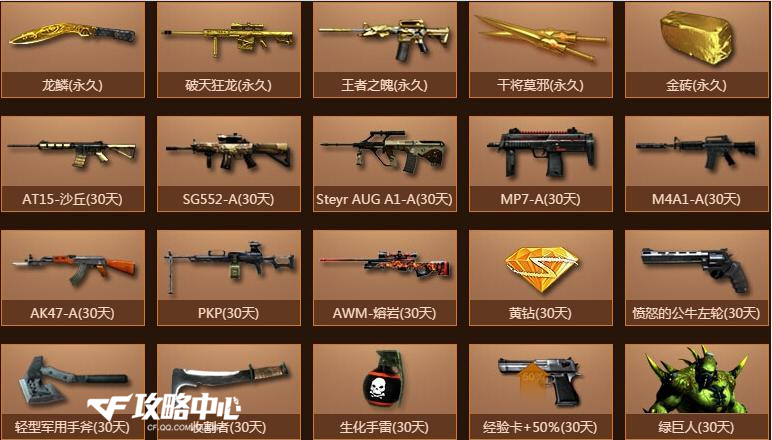\r

\r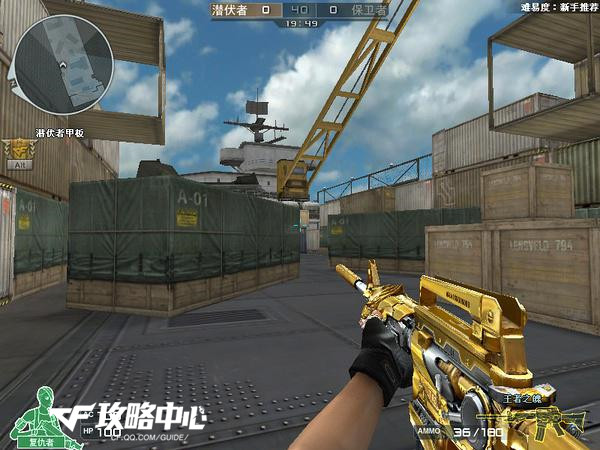\r

\r

\r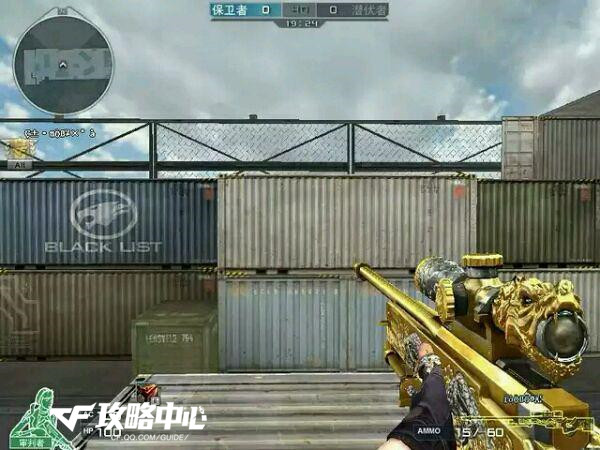\r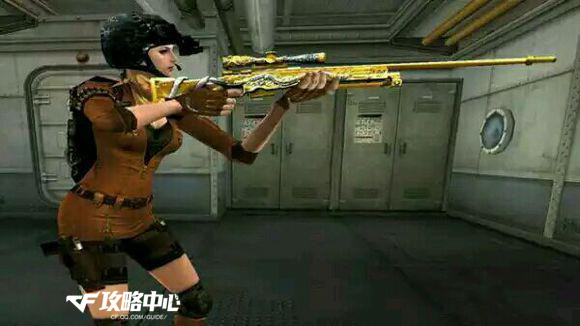\r

\r

\r

\r

\r

\r

\r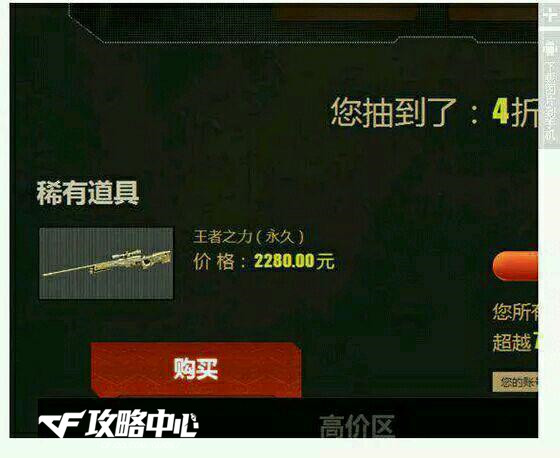\r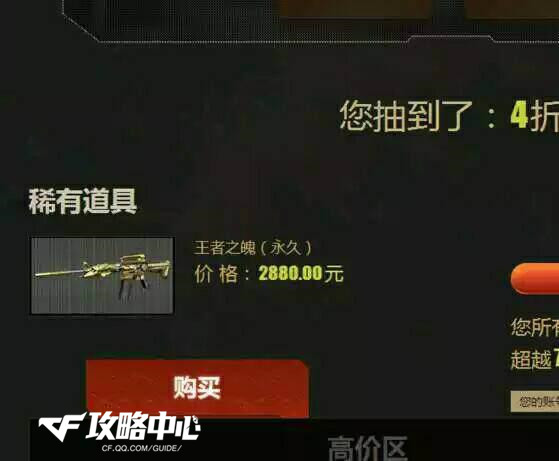\r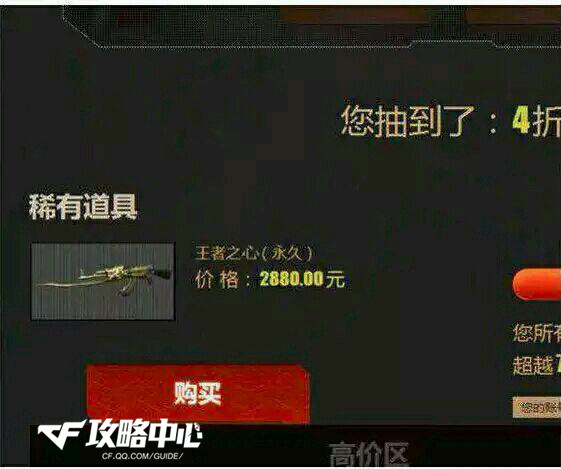\r

\r

\r

\r

\r

2015年我们迎来了挑战模式的续作——守卫挑战，塔防型的规则让不少Cfer都有了全新的体验，每回合的奖励宝箱设置，胜利后还能再次开出宝箱，在最终宝箱中，竟然出现了永久武器。

\r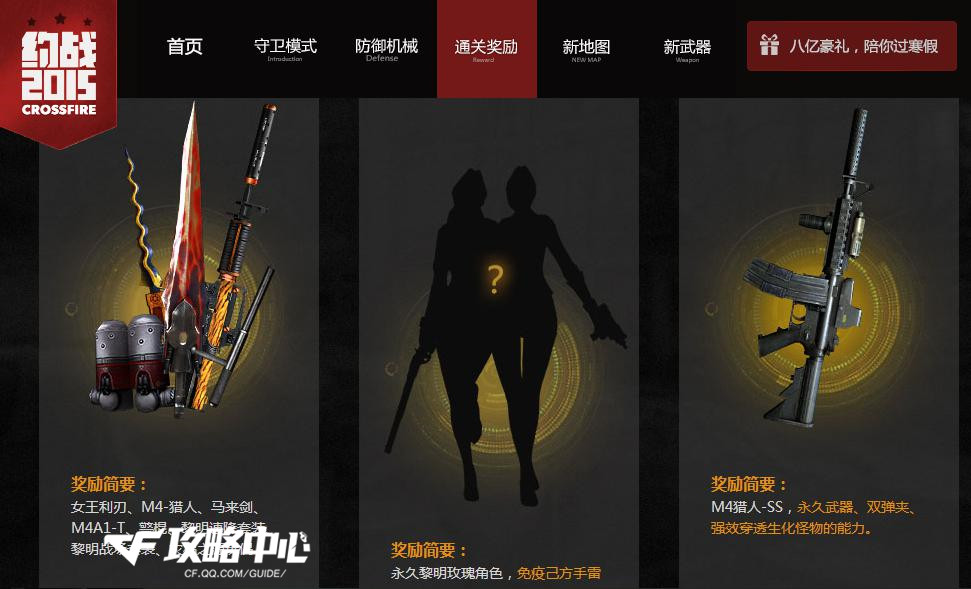\r

\r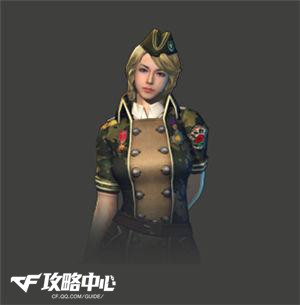\r

\r

\r

\r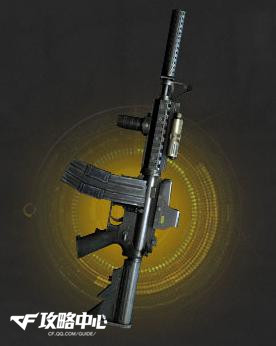\r

\r

\r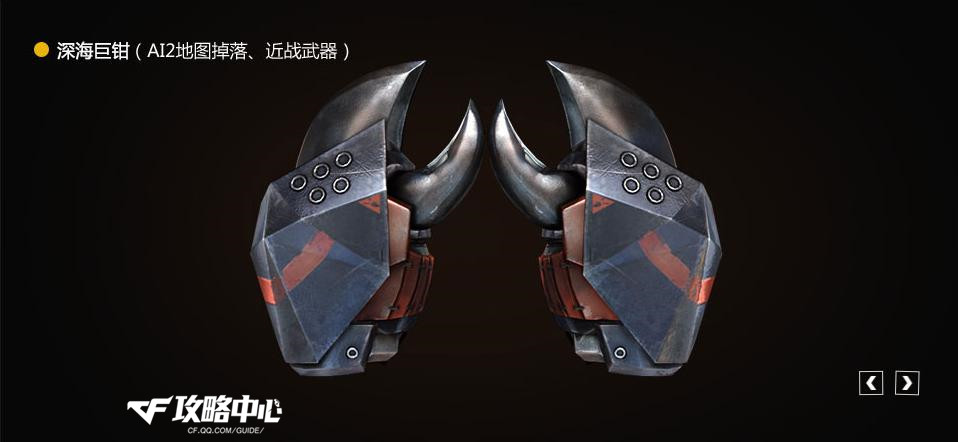\r

\r

\r

\r

\r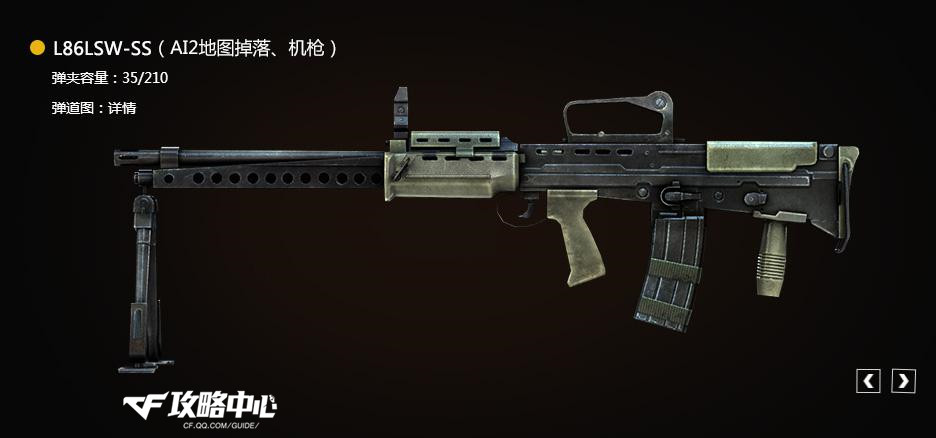\r

\r

\r

\r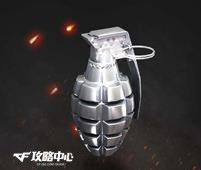\r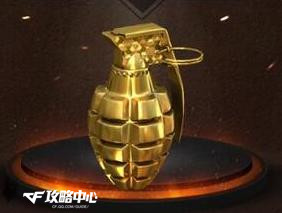\r

\r

\r

\r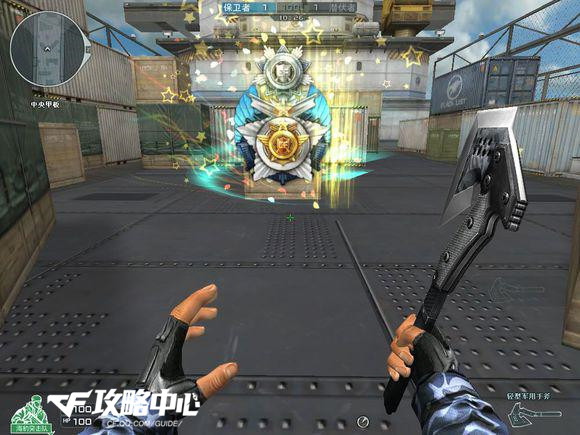\r

\r

\r

\r

AWM-A-枪王排位Ⅱ，AK47-枪王排位Ⅱ，M4A1-枪王排位

\r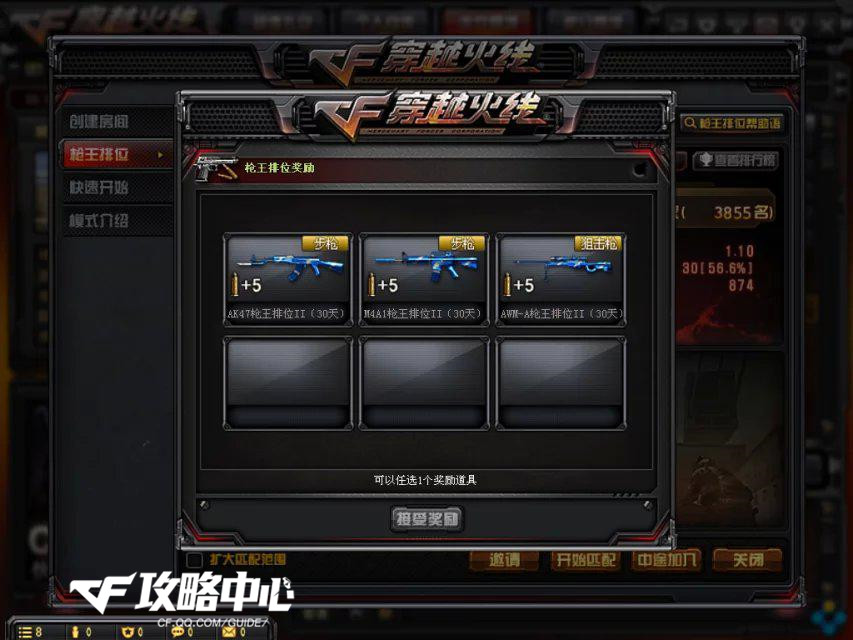\r

\r

\r

\r

\r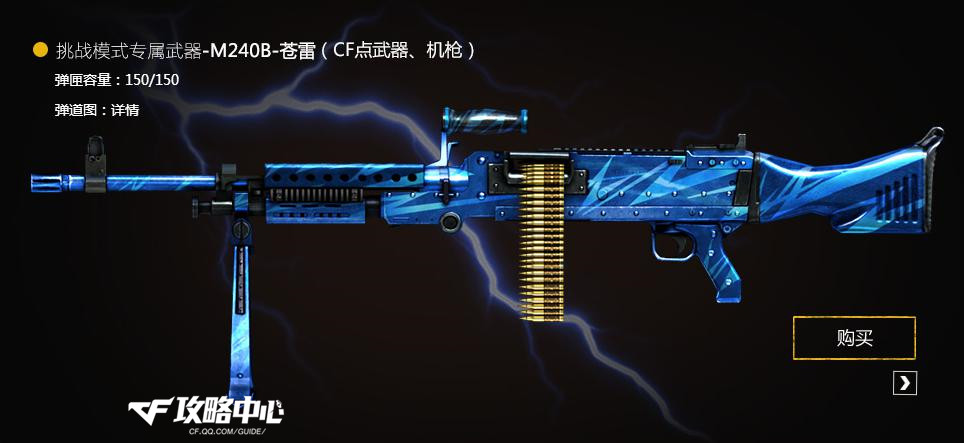\r

\r

179QB的低廉预售价让不少玩家大跌眼镜，拥有超越盘龙的性能，却只有盘龙的二分之一的价格。爽快的打击感，炫目的雷电子弹特效，三发一暴击的强大输出，苍雷炮的清场能力，综合起来，苍雷可谓是挑战武器中的王者。

\r

\r

\r

\r

?

\r

?

[www.tanseo.net]